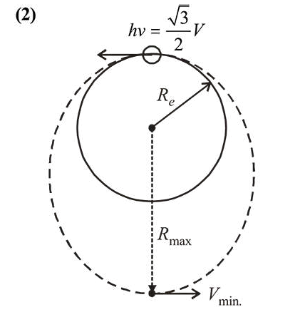# A satellite is moving in a low nearly circular orbit around the earth.Question:

A satellite is moving in a low nearly circular orbit around the earth. Its radius is roughly equal to that of the earth's radius $R_{e}$. By firing rockets attached to it, its speed is instantaneously increased in the direction of its motion so

that it become $\sqrt{\frac{3}{2}}$ times larger. Due to this the farthest

distance from the centre of the earth that the satellite reaches is $R$. Value of $R$ is :

1. (1) $4 R_{e}$

2. (2) $2.5 R_{e}$

3. (3) $3 R_{e}$

4. (4) $2 R_{e}$

Correct Option: , 2

Solution:Orbital velocity, $V_{0}=\sqrt{\frac{G M}{R_{e}}}$

From energy conversation,

$-\frac{G M m}{R_{e}}+\frac{1}{2} m\left(\sqrt{\frac{3}{2}} V\right)^{2}=\frac{G M m}{R_{\max }}+\frac{1}{2} m V_{\min }^{2}$     ....(1)

From angular momentum conversation

$\sqrt{\frac{3}{2}} V R_{e}=V_{\min } R_{\max }$

Solving equation (1) and (2) we get,

$R_{\max }=3 R_{e}$